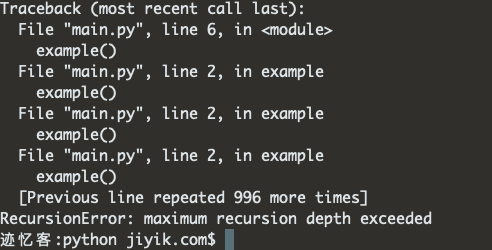# 迹忆客专注技术分享

## python 错误 RecursionError: maximum recursion depth exceeded

Python“RecursionError: maximum recursion depth exceeded”错误发生在函数被调用多次以致调用超过递归限制时。 要解决该错误，需要指定退出递归或设置更高递归限制必须满足的基本情况。``````def example():
example()

# ⛔️ RecursionError: maximum recursion depth exceeded
example()
``````

``````import sys

# 👇️ 1000
print(sys.getrecursionlimit())

# 👇️ set recursion limit to 2000
sys.setrecursionlimit(2000)

# 👇️ 2000
print(sys.getrecursionlimit())
``````

`getrecursionlimit` 方法返回 Python 解释器堆栈的最大深度。

``````counter = 0

def example(num):
global counter

if num < 0:
return # 👈️ 这会阻止函数无休止地调用自己

counter += 1
example(num - 1)

example(3)

print(counter)  # 👉️ 4
``````

``````def do_math(a, b):
return a + b

while True:
result = do_math(10, 10)
print(result)
``````

``````def do_math(a, b):
return a + b

total = 0
i = 10

while i > 0:
total += do_math(5, 5)

i = i - 1

print(total)  # 👉️ 100
``````## 热门标签